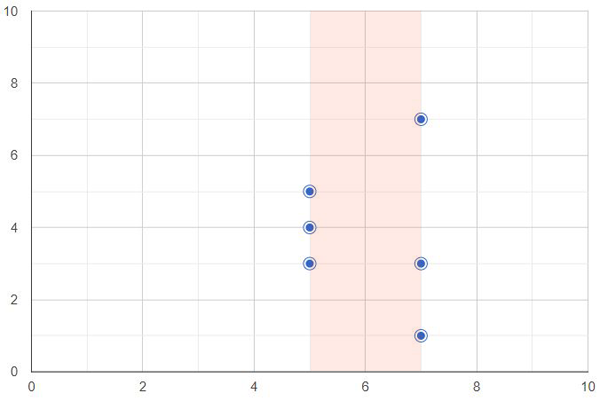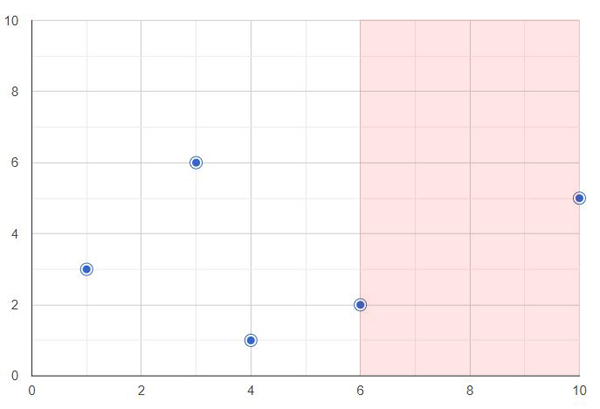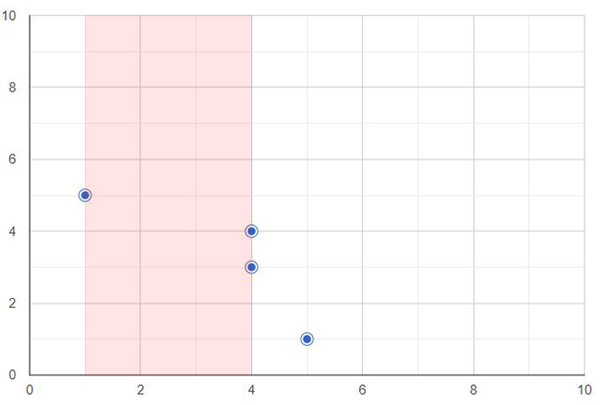# Microsoft Online Assessment (OA) - Widest Path Without Trees

There are `N` trees (numbered from `0` to `N-1`) in a forest. The `K-th` tree is located at coordinates `(X[K], Y[K])`. We want to build the widest possible vertical path, such that there is no tree on it. The path must be built somewhere between a leftmost and a rightmost tree, which means that the width of the path cannot be infinite.

What is the width of the widest possible path that can be built?

Write a function:

``1def solution(X, Y)``

that, given two arrays `X` and `Y` consisting of `N` integers each, denoting the positions of trees, returns the widest possible path that can be built.

#### Example 1:

Input: X = `[5,5,5,7,7,7]`, Y=`[3,4,5,1,3,7]`

Output: `2`#### Example 2:

Input: X = `[6,10,1,4,3]`, Y=`[2,5,3,1,6]`

Output: `4`#### Example 3:

Input: X = `[4,1,5,4]`, Y=`[4,5,1,3]`

Output: `3`## Implementation

``````1from typing import List
2
3def widest_path(x: List[int], y: List[int]) -> int:
4    difference = 0
5    sortedX = sorted(x)
6    for i in range(0, len(sortedX) - 1):
7        diff = sortedX[i + 1] - sortedX[i]
8        if diff > difference:
9            difference = diff
10    return difference
11
12if __name__ == '__main__':
13    x = [int(x) for x in input().split()]
14    y = [int(x) for x in input().split()]
15    res = widest_path(x, y)
16    print(res)
17``````